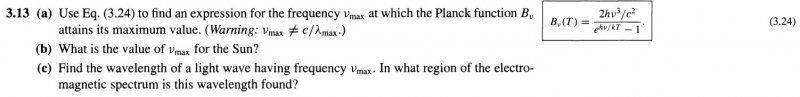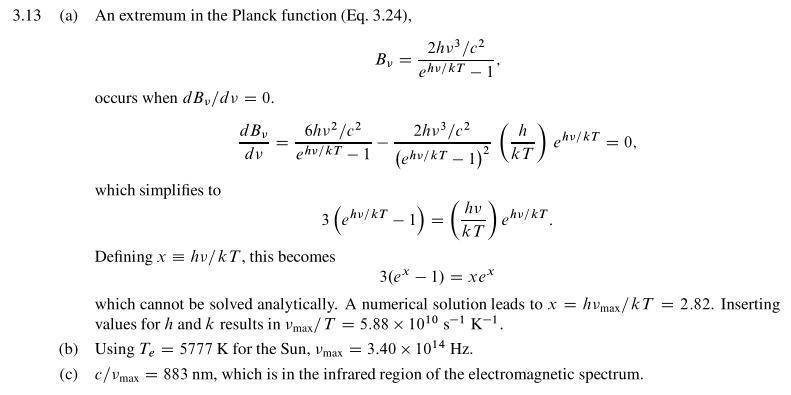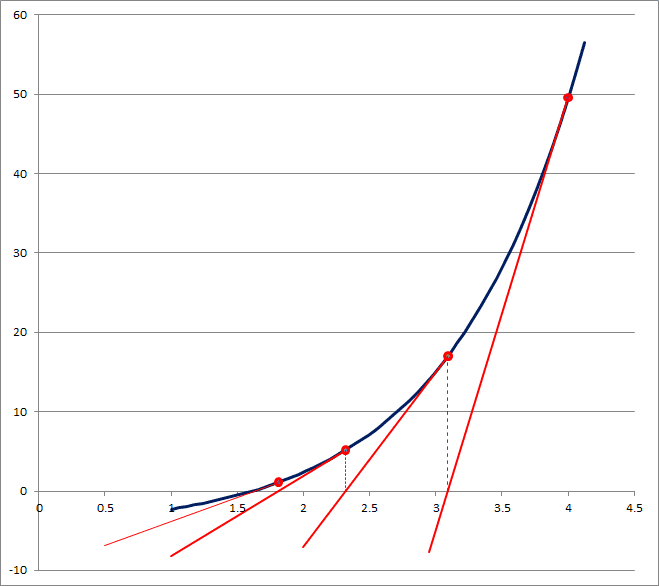# How to numerically solve an unsolvable equation

I'll include two pictures; the first one is the question from the book and the second one is the answer from the solution manual. I have no idea how they got that answer. For part a, the solution says that you can't solve it analytically, so you have to solve it numerically. What does that mean? You just make up numbers to put in there? There is no value for T to put in and no value for Vmax to put in, yet they somehow get 2.82 as the answer. To get that answer, for part a, they must have put in a value for Vmax, which is what we're trying to solve for, and put in a value for T, which has no value yet.Chestermiller
Mentor
Are you comfortable with the derivation of the third equation, which is the condition that has to be satisfied in order for the derivative of B with respect to ν to be equal to zero (for any arbitrary value of T)?

Chet

•leroyjenkens
Yeah, I derived all the way to the end on my own without using the solution manual, and got the same result they did. But after looking at it, it doesn't look like they actually did input numbers for V and T, which makes me wonder where the 2.82 came from. I tried solving for x, with x alone on the right side, and putting in various values for the x on the left side (the x's that are in the exponents of e), and it seems to approach 3 the higher I go. I don't know what that means, though.

Chestermiller
Mentor
You don't need to input numbers for ν and T. If you look at the third equation carefully, you will see that the same combination of parameters appears in all three places in the equation. They define this combination of parameters as x. Once you know that value of x that satisfies the equation, you can determine the value of ν that makes B maximum for any arbitrary value of T. That value value of ν is given by:
$$ν_{max}=\frac{kT}{h}x$$
To find the value of x, you need to solve the non-linear algebraic equation involving x. I can see that you started trying to solve the equation by using a so-called successive substitution approach, which was a good idea. But, not all successive substitution algorithms converge to a solution. Some of them diverge, and, the one you chose obviously diverges. The usual way of solving a non-linear algebraic equation like this is to apply Newton's Method. Are you familiar with Newton's Method?

Chet

•leroyjenkens
I wasn't aware of that, but I looked it up and tried to do it.
I graphed the equation in wolframalpha and it gave me a graph that intersects the x-axis at (0,0) and at what looks like (2.8,0). I then took the derivative of the 3rd equation by putting everything on the left side of the equation, leaving 0 on the right side, used that in the Newton's Method formula, input 2.8 in place of x, and got an answer of 2.71215. Is that the value of x?
Thanks for the response.
edit: Ok, I jumped the gun a little. So that's the first iteration. Since 2.8 and 2.71215 aren't the same, that means I need to do it again, using 2.71215 instead of 2.8. I'll post the results in an edit.
edit: Ok, I just did that and my next iteration was 2.404, which is getting farther away from 2.8, which I don't think is supposed to happen.

Last edited:
Chestermiller
Mentor
I wasn't aware of that, but I looked it up and tried to do it.
I graphed the equation in wolframalpha and it gave me a graph that intersects the x-axis at (0,0) and at what looks like (2.8,0). I then took the derivative of the 3rd equation by putting everything on the left side of the equation, leaving 0 on the right side, used that in the Newton's Method formula, input 2.8 in place of x, and got an answer of 2.71215. Is that the value of x?
Thanks for the response.
edit: Ok, I jumped the gun a little. So that's the first iteration. Since 2.8 and 2.71215 aren't the same, that means I need to do it again, using 2.71215 instead of 2.8. I'll post the results in an edit.
edit: Ok, I just did that and my next iteration was 2.404, which is getting farther away from 2.8, which I don't think is supposed to happen.

Chet

•leroyjenkens

Chet
Ok, I'm redoing it because I made a mistake deriving it, but here is the Newton's Method formula I'm using:
$$X_{n+1}=X_{n}-\frac{f(X_{n})}{f'{(X_{n})}}$$

Here is my
$$f(X_{n}) = 3(e^{x}-1)-xe^{x}$$

And my
$$f'(X_{n}) = 2e^{x}-xe^{x}$$

After doing it again with the correct derivative, I got 2.8219 as the answer for x, which is pretty close to the starting 2.8. If it repeats, then that will be my value for x.

Ok, after continually doing it, it's converging to 2.82. Seems like this worked, thanks a lot.

Last edited:
Chestermiller
Mentor
Ok, I'm redoing it because I made a mistake deriving it, but here is the Newton's Method formula I'm using:
$$X_{n+1}=X_{n}-\frac{f(X_{n})}{f'{(X_{n})}}$$

Here is my
$$f(X_{n}) = 3(e^{x}-1)-xe^{x}$$

And my
$$f'(X_{n}) = 2e^{x}-xe^{x}$$

After doing it again with the correct derivative, I got 2.8219 as the answer for x, which is pretty close to the starting 2.8. If it repeats, then that will be my value for x.
It will. Very nicely done!

Chet

•leroyjenkens
Thanks, you've been a big help.

SteamKing
Staff Emeritus
Homework Helper
For part a, the solution says that you can't solve it analytically, so you have to solve it numerically. What does that mean? You just make up numbers to put in there?

Pretty much. There are plenty of equations whose solutions cannot be derived algebraically.

The essence of an iterative solution is that a trial value is inserted into the equation and the equation is then evaluated. There will be a numerical disagreement between the LHS and the RHS of the equation in all probability. Another trial value, hopefully a refinement of the previous trial value, is inserted and the process repeats, until the numerical disagreement between the LHS and the RHS of the equation is less than some arbitrary tolerance.

Hydraulics and friction due to fluid flow are two examples of fields where iterative solutions to problems are used quite a bit.

Real science and engineering is often messy like that. It's a far cry from algebra class where the solutions to an equation fall out all nice and neat. :)

I wasn't aware of that, but I looked it up and tried to do it.
edit: Ok, I just did that and my next iteration was 2.404, which is getting farther away from 2.8, which I don't think is supposed to happen.

Just to clarify this, Each iteration of Newton's method alternates from a value that is greater than the zero to a value that is lesser than the value. This is because Newton's Method takes the input value (i.e., the guess) and finds out where the tangent line at x crosses the x-axis, which becomes the new guess. If you draw some arbitrary curve you will see (as you should intuit) that this gives the "alternating" answers. This method converges (i think guaranteed - albeit somewhat slowly) because the tangent line at the zero point clearly only intersects at the zero .

EDIT: Newton's method is also the method your typically TI calculator uses to find zeroes.

Chestermiller
Mentor
Just to clarify this, Each iteration of Newton's method alternates from a value that is greater than the zero to a value that is lesser than the value. This is because Newton's Method takes the input value (i.e., the guess) and finds out where the tangent line at x crosses the x-axis, which becomes the new guess. If you draw some arbitrary curve you will see (as you should intuit) that this gives the "alternating" answers. This method converges (i think guaranteed - albeit somewhat slowly) because the tangent line at the zero point clearly only intersects at the zero .
This is not correct. In the solution to a problem using Newton's Method, the error does not always alternate in sign. Just draw a few curves and apply Newton's method graphically, and you will see this. Also, the convergence is typically very rapid. For example, if the relationship is linear, Newton's Method converges in 1 iteration. As the solution is approached, the error from one iteration to the next decreases as the square of the distance to the solution. This is called second order convergence.

Chet

BvU
Homework Helper
Just to clarify this, Each iteration of Newton's method alternates from a value that is greater than the zero to a value that is lesser than the value. This is because Newton's Method takes the input value (i.e., the guess) and finds out where the tangent line at x crosses the x-axis, which becomes the new guess. If you draw some arbitrary curve you will see (as you should intuit) that this gives the "alternating" answers. This method converges (i think guaranteed - albeit somewhat slowly) because the tangent line at the zero point clearly only intersects at the zero .

EDIT: Newton's method is also the method your typically TI calculator uses to find zeroes.
Hello Delcross, welcome to PF :)

A small rectification: Newton is not a root bracketing method (like bisection or regula falsi)

Simple counter-example: ##f(x) = e^x - 5## starting from ##x_0## = 4:
Code:
Iter          x                     f(x)

0           4                   49.60
1           3.092               17.01
2           2.319                5.16
3           1.811                1.114860
4           1.628                0.09573
5           1.609616601          0.00089
6           1.609437928          8.0E-08

ln(5)       1.609437912Regula Falsi and secant methods amount to NR equivalents but with (initially coarse) numerical derivatives (so they end up in digital noise if you're not careful).

Newton converges very rapidly once going (roughly doubles the number of significant digits per iteration). If it converges. Contra-indications are: wrong sign of ##f'## or (even worse) ##f'(x_0)=0##. So you want to have good derivatives and start near a solution. Maybe by starting off with one of the other, more robust methods.

Last edited:
•Chestermiller
Also, the convergence is typically very rapid. For example, if the relationship is linear, Newton's Method converges in 1 iteration. As the solution is approached, the error from one iteration to the next decreases as the square of the distance to the solution. This is called second order convergence.
Chet

Thanks for correcting about bracketing. But, Newton's method is not always quadratically convergent and has issues with multiple roots. It can be modified to include second derivatives to regain its quadratic convergence, but even then you also add many more steps to perform each iteration. Other methods such as Steffenson's method give you quadratic convergence without having to calculate any derivatives.

BvU
•